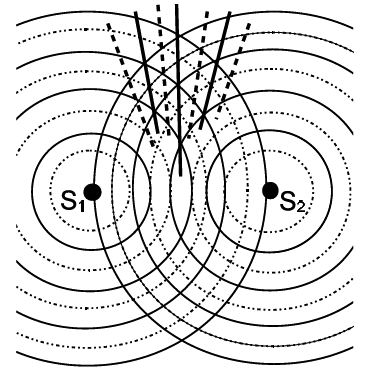# Difference between Interference and Diffraction

Two separate wavefronts originating from two coherent sources produce interference. Secondary wavelets originating from dissimilar parts of the similar wavefront constitute diffraction. The vital difference between diffraction and interference is that diffraction of light occurs due to the superposition of secondary wavelets that generates from various parts of a wavefront. Another significant difference between the two is that the intensity of all the bright fringes is not similar in the case of diffraction however it is similar in case of interference.

Difference between interference and diffraction:

Interference

• Interference is the result of the interaction of two different wavefronts originating from the same source. It is due to the interaction of light coming from two different wavefronts originating from the same source.
• It is due to the superposition of secondary wavelets from two different wavefronts produced by two coherent sources.
• Fringes are equally spaced. The width of the fringes is equal in interference.
• Bright fringes are of the same intensity. When we have two infinitely narrow slits separated by a distance apart near the source, we get interference.
• Comparing with diffraction, it has a large number of fringes
• All bright bands are of uniform intensity.
• The region of minimum intensity is perfectly dark in interference.
• In interference, the intensity of all the positions on maxima is of similar intensity in interference.
• If the number of sources is few such as two sources, then they are referred to as interference sources.

Diffraction

• Diffraction is the result of interaction from different parts of the same wavefront. It is due to the interaction of light coming from different parts of the same wavefront.
• It is due to the superposition of secondary wavelets emitted from various points of the same wavefront.
• Fringes are unequally spaced. In diffraction, the width of the fringes is never equal.
• Intensity falls rapidly. When we have a single slit of finite width or rather an aperture near the source, we get diffraction.
• It has less number of fringes
• All bright bands are not of the same intensity.
• In diffraction, the region of minimum intensity is not perfectly dark.
• In diffraction, there is a variance in the intensity of positions.
• If the number of sources is many, that is more than two then it is referred to as diffraction sources.